## Properties of Basic Mathematical Operations

Some mathematical operations have properties that can make them easier to work with and actually can save you time.

#### Some properties (axioms) of addition

• Closure is when all answers fall into the original set. If you add two even numbers, the answer is still an even number (2 + 4 = 6); therefore, the set of even numbers is closed under addition (has closure). If you add two odd numbers, the answer is not an odd number (3 + 5 = 8); therefore, the set of odd numbers is not closed under addition (no closure).
• Commutative means that the order does not make any difference in the operation's result.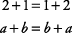• Note: Commutative does not hold for subtraction.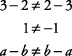• Associative means that the grouping does not make any difference in the operation's result.The grouping has changed (parentheses moved), but the sides are still equal.
• Note: Associative does not hold for subtraction.• The identity element for addition is 0.Any number added to 0 gives you the original number.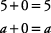• The additive inverse is the opposite (negative) of the number. Any number plus its additive inverse equals 0 (the identity).
3 + (–3) = 0; therefore, 3 and –3 are additive inverses.
–4 + 4 = 0; therefore, –4 and 4 are additive inverses.
a + (– a) = 0; therefore, a and – a are additive inverses.

#### Some properties (axioms) of multiplication

• Closure is when all answers fall into the original set. If you multiply two even numbers, the answer is still an even number (2 × 4 = 8); therefore, the set of even numbers is closed under multiplication (has closure). If you multiply two odd numbers, the answer is an odd number (3 × 5 = 15); therefore, the set of odd numbers is closed under multiplication (has closure).
• Commutative means that the order does not make any difference in the operation's result.Note: Commutative does not hold for division.• Associative means that the grouping does not make any difference in the operation's result.The grouping has changed (parentheses moved), but the sides are still equal.

Note: Associative does not hold for division.• The identity element for multiplication is 1. Any number multiplied by 1 gives the original number.• The multiplicative inverse is the reciprocal of the number. Any nonzero number multiplied by its reciprocal equals 1.; therefore, 2 andare multiplicative inverses, or reciprocals.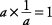; therefore, a and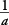are multiplicative inverses, or reciprocals (provided a ≠ 0).

#### A property of two operations

The distributive property is the process of distributing, using multiplication, the number on the outside of the parentheses to each term on the inside. The terms within the parentheses are separated by either addition or subtraction.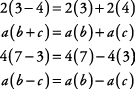Note: You cannot use the distributive property with only one operation.